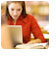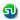### TANCET 2008 Question and solution : Framing Equations : Ascent Education TANCET MBA Classes

 Home TANCET Classes GMAT Coaching Online GMAT Course CAT Classes TANCET Study Material
 TANCET '18 Classroom ProgramWeekend and weekday classes for TANCET MBA @ Chennai. At Nungambakkam and Velachery.Other Courses TANCET Correspondence Course CAT Classes & Courses GMAT Classes Chennai Online GMAT Course GRE Classes Chennai CBSE Math Online Tuition SAT Classes Other Links Ascent TANCET Toppers Testimonials CAT, TANCET Questions Careers @ Ascent Contact Us +91 44 4500 8484 +91 96000 48484 ascent@ascenteducation.com Postal Address Facebook / Twitter / Blog / Videos
You are here: Home  »  TANCET Prep Questions »  Linear Equations »   TANCET 2008 Question 2

# TANCET 2008 Quant Question 2 : Framing Equation

## Question

Jack has three more cards than Bill. Together they have 47 cards. If x represents the number of cards Bill has, then an equation that can be used to determine the number of cards each one has is
1. x + 3 = 47
2. 2x + 3 = 47
3. x - 3 = 47
4. 2x - 3 = 47
5. 3x + 3 = 47

Correct Answer : 2x + 3 = 47. Choice (2)

Bill has x cards.

Jack has 3 more cards than Bill. So, Jack has x + 3 cards.

So, together Bill and Jack have x + x + 3 = 2x + 3 cards.

The question states that they have 47 cards between them.

So, 2x + 3 = 47.

Level of difficulty : Very Easy

## More Questions on Linear, Quadratic and Cubic Equations - Algebra

 5. 6. 7.

## CAT, XAT, TANCET Practice Questions and Answers : Listed Topicwise

 Number Theory Permutation Combination Probability Inequalities Geometry Mensuration Trigonometry Coordinate Geometry Percentages Profit Loss Ratio Proportion Mixtures Alligation Speed Time Distance Pipes Cisterns Interest Races Average, Mean AP, GP, HP Set Theory Clocks Calendars Algebra Function English Grammar General Awareness Data sufficiency TANCET Papers XAT PapersAdd to del.icio.usStumble Itdigg this